# RD Sharma Solutions for Class 9 Maths Chapter 6 Factorization of Polynomials Exercise 6.5

RD Sharma Class 9 Mathematics Chapter 6 Exercise 6.5 Factorization of Polynomials is provided here. In this exercise, students will learn how to perform factorisation of a polynomial with integral coefficient using factor theorem. The solutions covered in RD Sharma Class 9 are detailed with easy explanations so that the students can easily comprehend and understand the concepts in detail.

## Download PDF of RD Sharma Solutions for Class 9 Maths Chapter 6 Factorization of Polynomials Exercise 6.5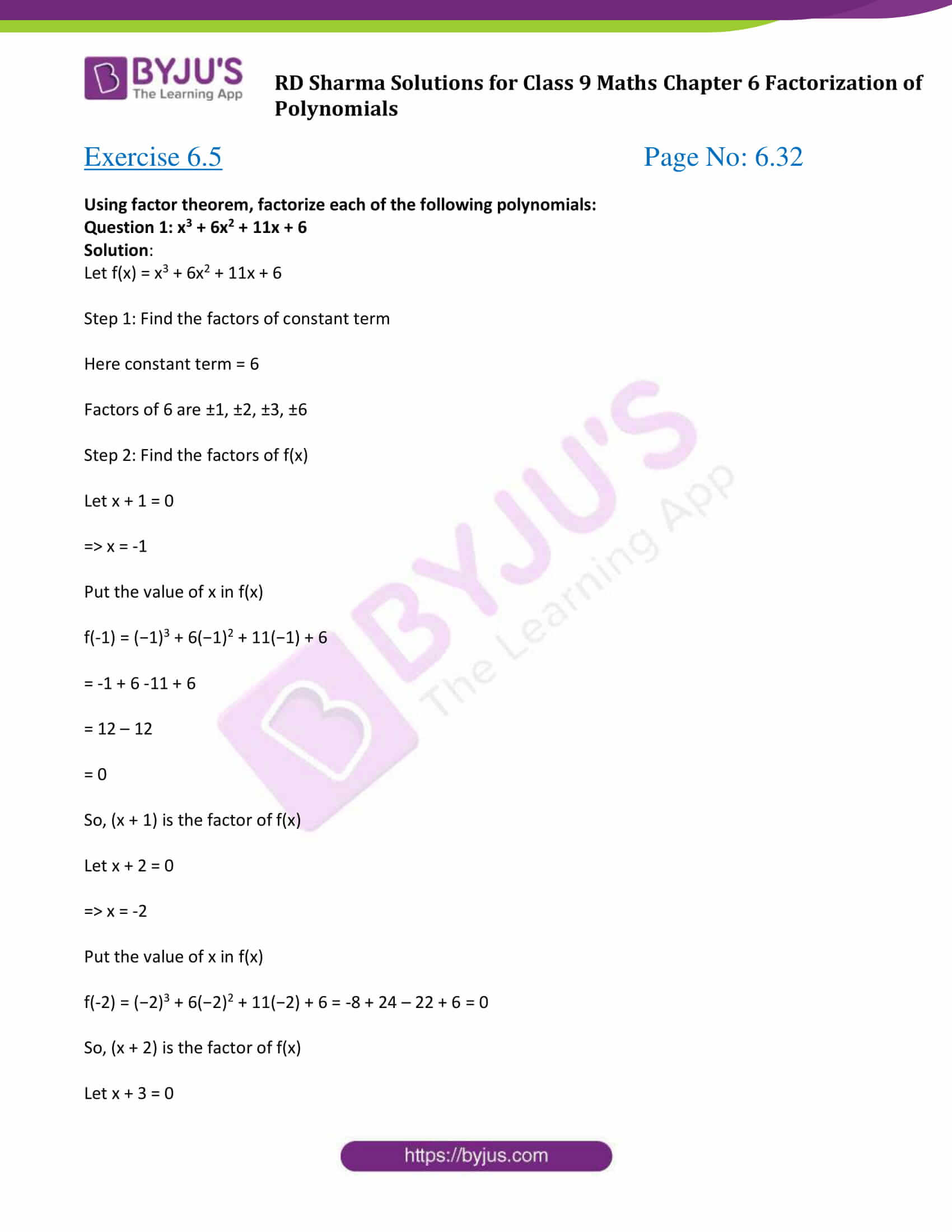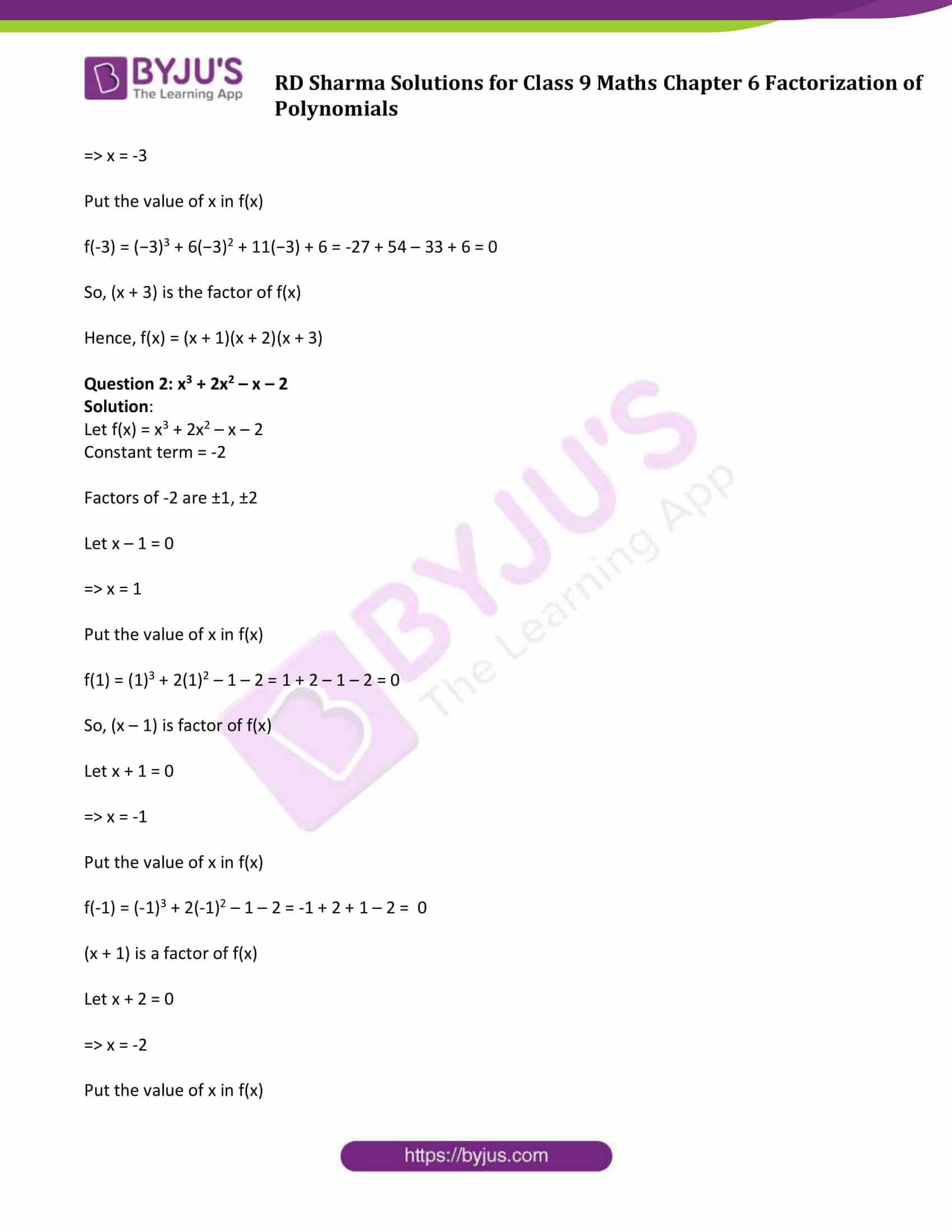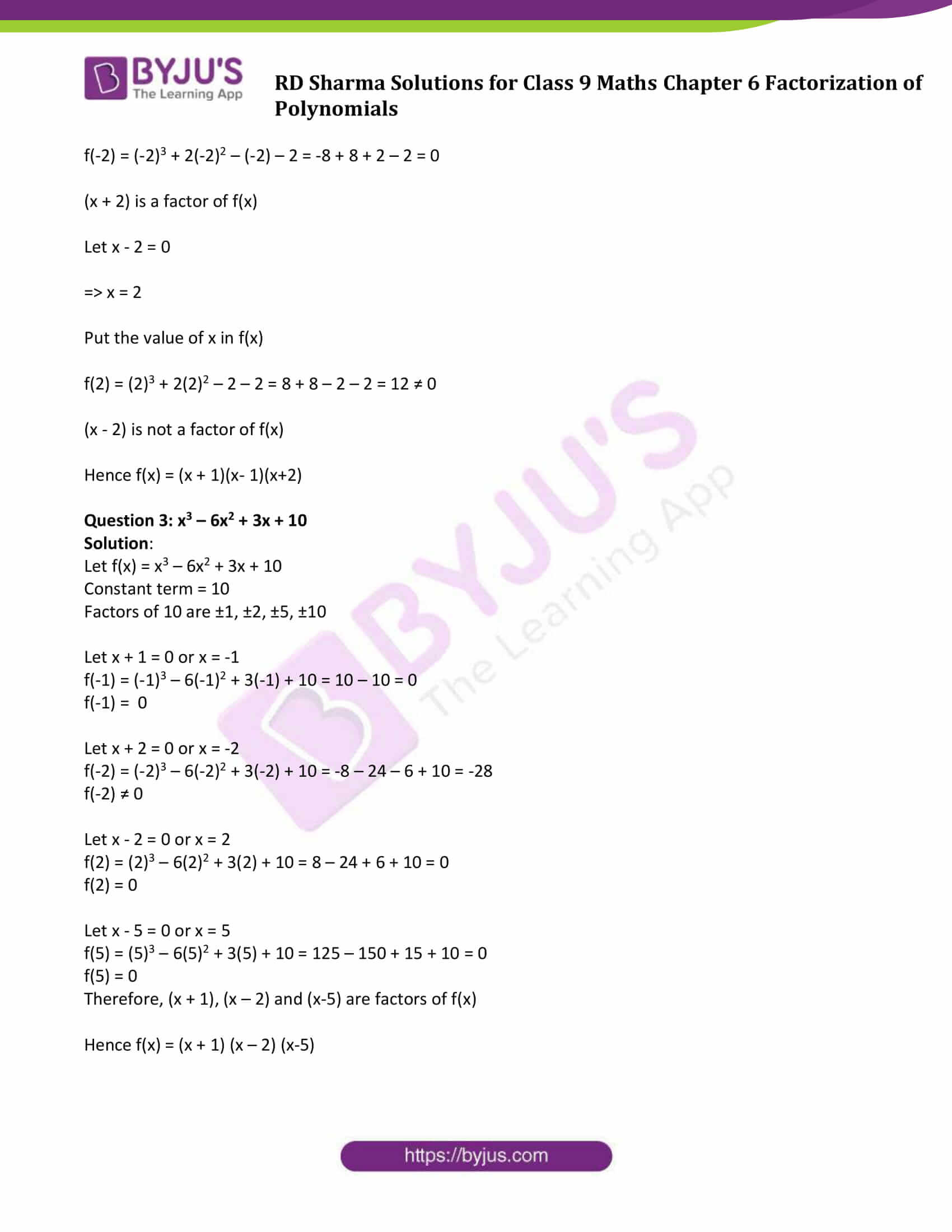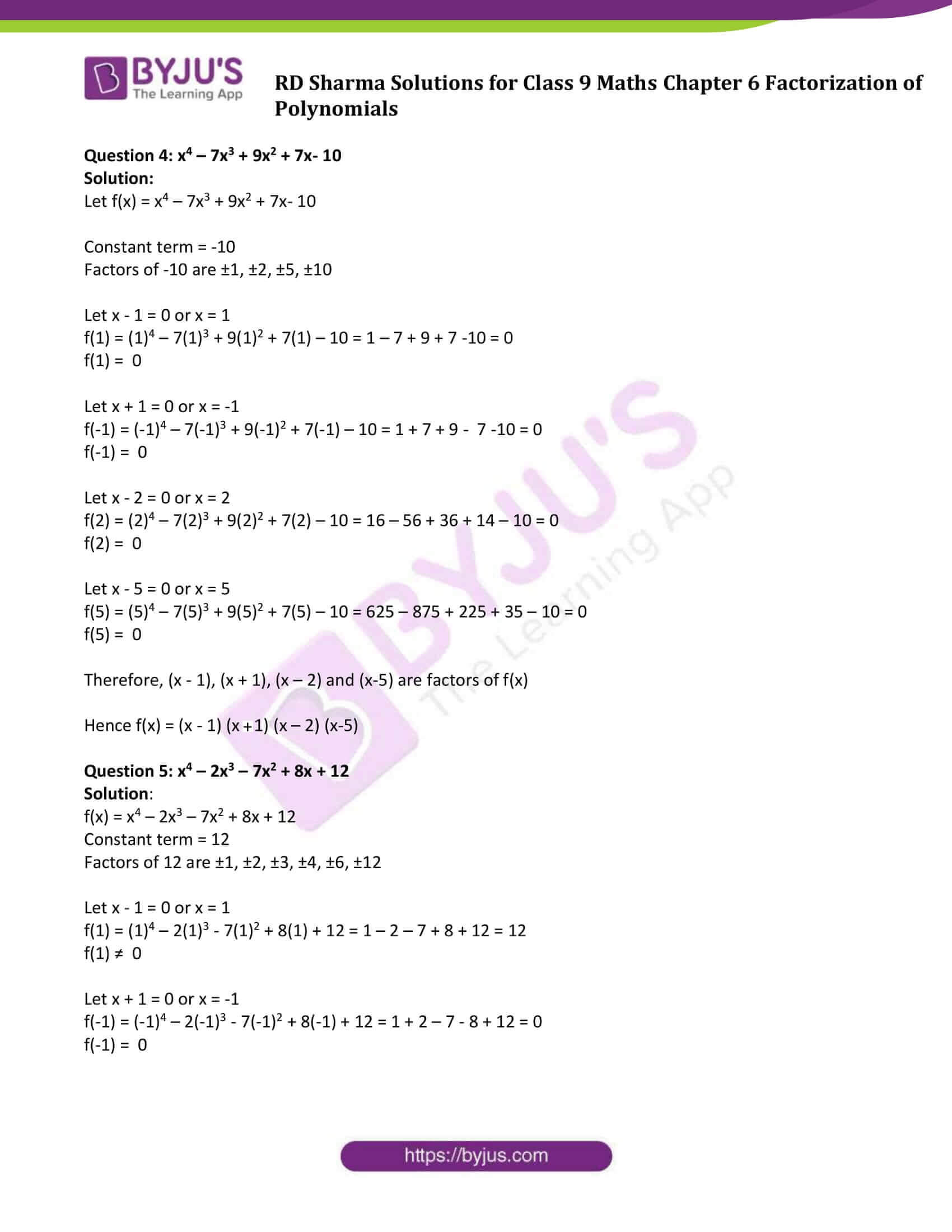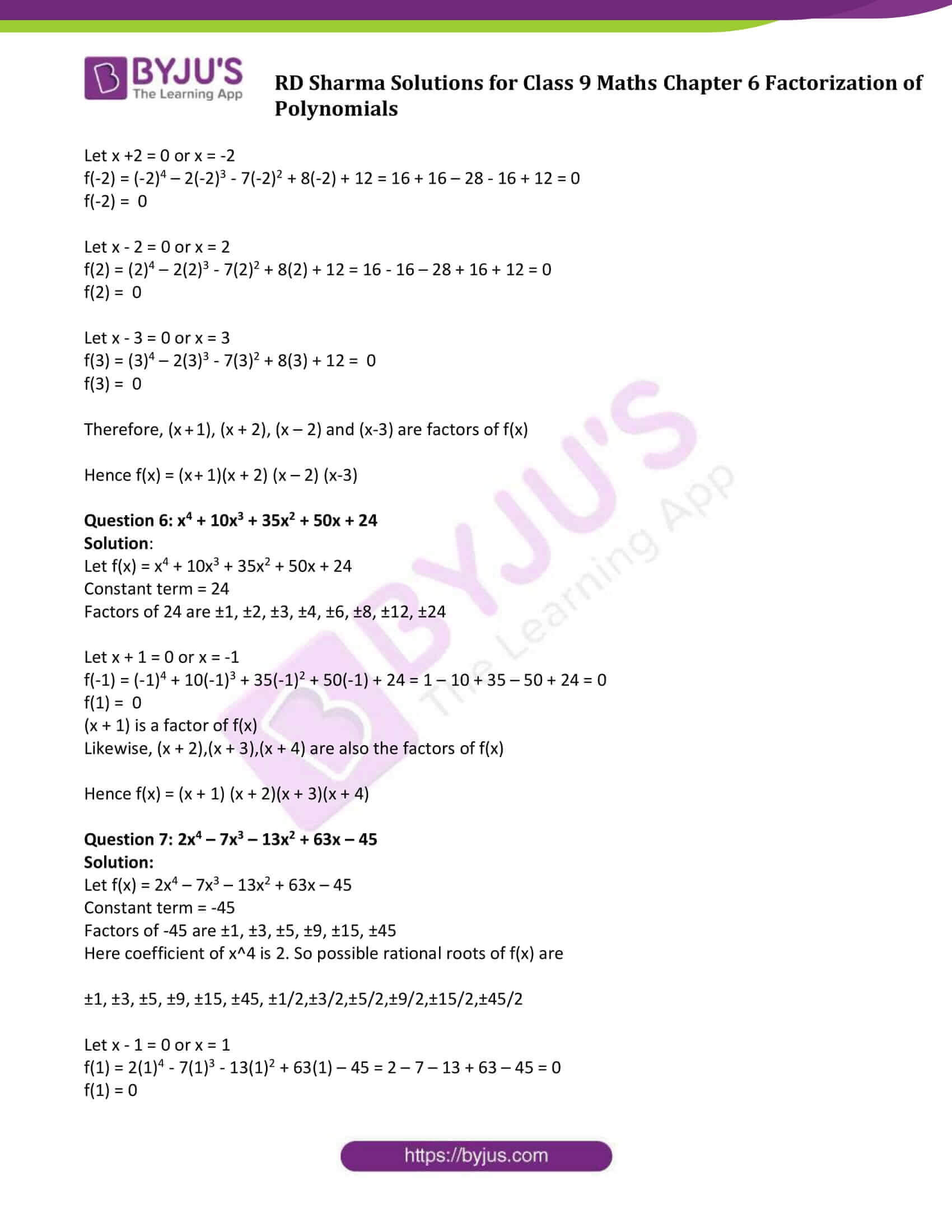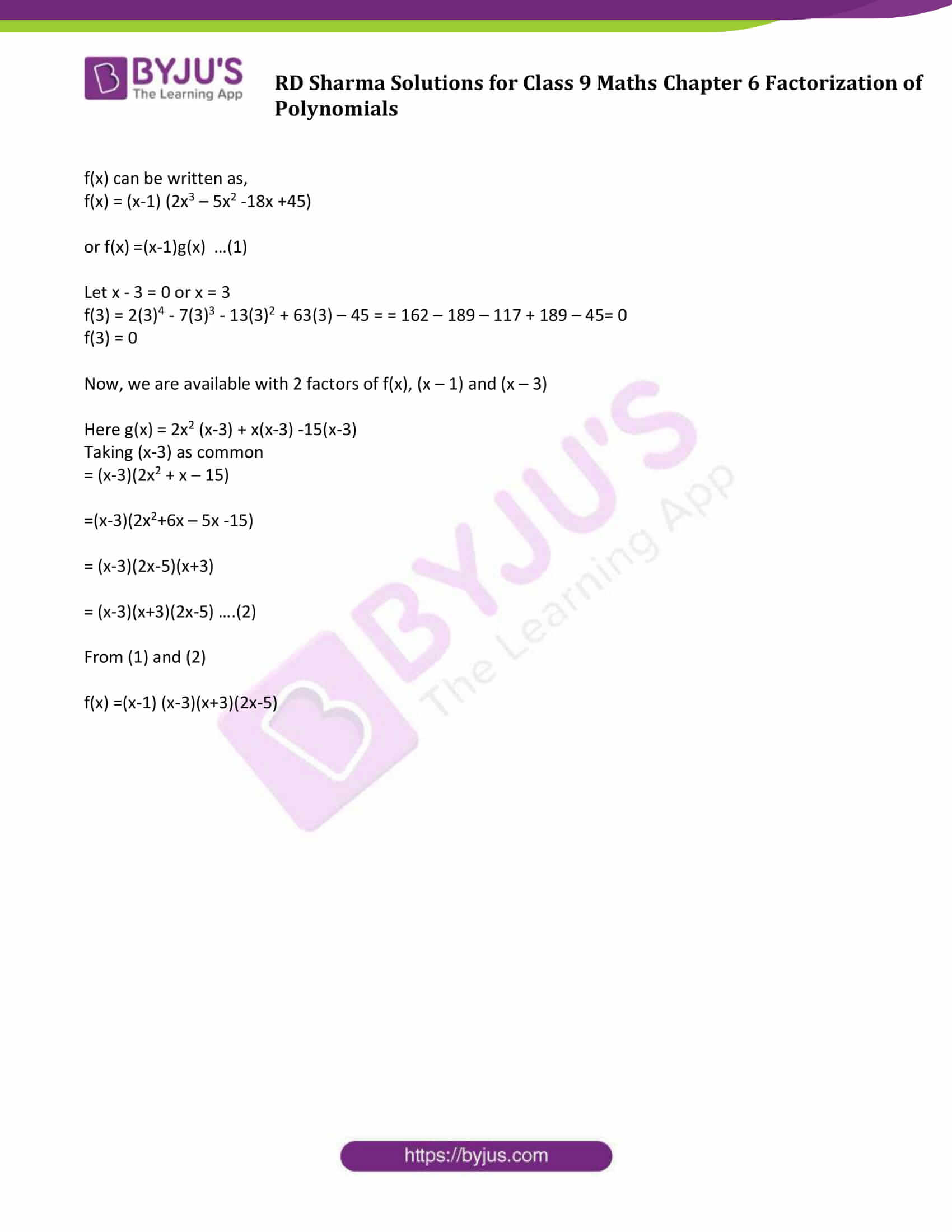### Access Answers to Maths RD Sharma Solutions for Class 9 Chapter 6 Factorization of Polynomials Exercise 6.5 Page number 6.32

#### Exercise 6.5 Page No: 6.32

Using factor theorem, factorize each of the following polynomials:

Question 1: x3 + 6x2 + 11x + 6

Solution:

Let f(x) = x3 + 6x2 + 11x + 6

Step 1: Find the factors of constant term

Here constant term = 6

Factors of 6 are ±1, ±2, ±3, ±6

Step 2: Find the factors of f(x)

Let x + 1 = 0

⇒ x = -1

Put the value of x in f(x)

f(-1) = (−1)3 + 6(−1)2 + 11(−1) + 6

= -1 + 6 -11 + 6

= 12 – 12

= 0

So, (x + 1) is the factor of f(x)

Let x + 2 = 0

⇒ x = -2

Put the value of x in f(x)

f(-2) = (−2)3 + 6(−2)2 + 11(−2) + 6 = -8 + 24 – 22 + 6 = 0

So, (x + 2) is the factor of f(x)

Let x + 3 = 0

⇒ x = -3

Put the value of x in f(x)

f(-3) = (−3)3 + 6(−3)2 + 11(−3) + 6 = -27 + 54 – 33 + 6 = 0

So, (x + 3) is the factor of f(x)

Hence, f(x) = (x + 1)(x + 2)(x + 3)

Question 2: x3 + 2x2 – x – 2

Solution:

Let f(x) = x3 + 2x2 – x – 2

Constant term = -2

Factors of -2 are ±1, ±2

Let x – 1 = 0

⇒ x = 1

Put the value of x in f(x)

f(1) = (1)3 + 2(1)2 – 1 – 2 = 1 + 2 – 1 – 2 = 0

So, (x – 1) is factor of f(x)

Let x + 1 = 0

⇒ x = -1

Put the value of x in f(x)

f(-1) = (-1)3 + 2(-1)2 – 1 – 2 = -1 + 2 + 1 – 2 = 0

(x + 1) is a factor of f(x)

Let x + 2 = 0

⇒ x = -2

Put the value of x in f(x)

f(-2) = (-2)3 + 2(-2)2 – (-2) – 2 = -8 + 8 + 2 – 2 = 0

(x + 2) is a factor of f(x)

Let x – 2 = 0

⇒ x = 2

Put the value of x in f(x)

f(2) = (2)3 + 2(2)2 – 2 – 2 = 8 + 8 – 2 – 2 = 12 ≠ 0

(x – 2) is not a factor of f(x)

Hence f(x) = (x + 1)(x- 1)(x+2)

Question 3: x3 – 6x2 + 3x + 10

Solution:

Let f(x) = x3 – 6x2 + 3x + 10

Constant term = 10

Factors of 10 are ±1, ±2, ±5, ±10

Let x + 1 = 0 or x = -1

f(-1) = (-1)3 – 6(-1)2 + 3(-1) + 10 = 10 – 10 = 0

f(-1) = 0

Let x + 2 = 0 or x = -2

f(-2) = (-2)3 – 6(-2)2 + 3(-2) + 10 = -8 – 24 – 6 + 10 = -28

f(-2) ≠ 0

Let x – 2 = 0 or x = 2

f(2) = (2)3 – 6(2)2 + 3(2) + 10 = 8 – 24 + 6 + 10 = 0

f(2) = 0

Let x – 5 = 0 or x = 5

f(5) = (5)3 – 6(5)2 + 3(5) + 10 = 125 – 150 + 15 + 10 = 0

f(5) = 0

Therefore, (x + 1), (x – 2) and (x-5) are factors of f(x)

Hence f(x) = (x + 1) (x – 2) (x-5)

Question 4: x4 – 7x3 + 9x2 + 7x- 10

Solution:

Let f(x) = x4 – 7x3 + 9x2 + 7x- 10

Constant term = -10

Factors of -10 are ±1, ±2, ±5, ±10

Let x – 1 = 0 or x = 1

f(1) = (1)4 – 7(1)3 + 9(1)2 + 7(1) – 10 = 1 – 7 + 9 + 7 -10 = 0

f(1) = 0

Let x + 1 = 0 or x = -1

f(-1) = (-1)4 – 7(-1)3 + 9(-1)2 + 7(-1) – 10 = 1 + 7 + 9 – 7 -10 = 0

f(-1) = 0

Let x – 2 = 0 or x = 2

f(2) = (2)4 – 7(2)3 + 9(2)2 + 7(2) – 10 = 16 – 56 + 36 + 14 – 10 = 0

f(2) = 0

Let x – 5 = 0 or x = 5

f(5) = (5)4 – 7(5)3 + 9(5)2 + 7(5) – 10 = 625 – 875 + 225 + 35 – 10 = 0

f(5) = 0

Therefore, (x – 1), (x + 1), (x – 2) and (x-5) are factors of f(x)

Hence f(x) = (x – 1) (x + 1) (x – 2) (x-5)

Question 5: x4 – 2x3 – 7x2 + 8x + 12

Solution:

f(x) = x4 – 2x3 – 7x2 + 8x + 12

Constant term = 12

Factors of 12 are ±1, ±2, ±3, ±4, ±6, ±12

Let x – 1 = 0 or x = 1

f(1) = (1)4 – 2(1)3 – 7(1)2 + 8(1) + 12 = 1 – 2 – 7 + 8 + 12 = 12

f(1) ≠ 0

Let x + 1 = 0 or x = -1

f(-1) = (-1)4 – 2(-1)3 – 7(-1)2 + 8(-1) + 12 = 1 + 2 – 7 – 8 + 12 = 0

f(-1) = 0

Let x +2 = 0 or x = -2

f(-2) = (-2)4 – 2(-2)3 – 7(-2)2 + 8(-2) + 12 = 16 + 16 – 28 – 16 + 12 = 0

f(-2) = 0

Let x – 2 = 0 or x = 2

f(2) = (2)4 – 2(2)3 – 7(2)2 + 8(2) + 12 = 16 – 16 – 28 + 16 + 12 = 0

f(2) = 0

Let x – 3 = 0 or x = 3

f(3) = (3)4 – 2(3)3 – 7(3)2 + 8(3) + 12 = 0

f(3) = 0

Therefore, (x + 1), (x + 2), (x – 2) and (x-3) are factors of f(x)

Hence f(x) = (x + 1)(x + 2) (x – 2) (x-3)

Question 6: x4 + 10x3 + 35x2 + 50x + 24

Solution:

Let f(x) = x4 + 10x3 + 35x2 + 50x + 24

Constant term = 24

Factors of 24 are ±1, ±2, ±3, ±4, ±6, ±8, ±12, ±24

Let x + 1 = 0 or x = -1

f(-1) = (-1)4 + 10(-1)3 + 35(-1)2 + 50(-1) + 24 = 1 – 10 + 35 – 50 + 24 = 0

f(1) = 0

(x + 1) is a factor of f(x)

Likewise, (x + 2),(x + 3),(x + 4) are also the factors of f(x)

Hence f(x) = (x + 1) (x + 2)(x + 3)(x + 4)

Question 7: 2x4 – 7x3 – 13x2 + 63x – 45

Solution:

Let f(x) = 2x4 – 7x3 – 13x2 + 63x – 45

Constant term = -45

Factors of -45 are ±1, ±3, ±5, ±9, ±15, ±45

Here coefficient of x^4 is 2. So possible rational roots of f(x) are

±1, ±3, ±5, ±9, ±15, ±45, ±1/2,±3/2,±5/2,±9/2,±15/2,±45/2

Let x – 1 = 0 or x = 1

f(1) = 2(1)4 – 7(1)3 – 13(1)2 + 63(1) – 45 = 2 – 7 – 13 + 63 – 45 = 0

f(1) = 0

f(x) can be written as,

f(x) = (x-1) (2x3 – 5x2 -18x +45)

or f(x) =(x-1)g(x) …(1)

Let x – 3 = 0 or x = 3

f(3) = 2(3)4 – 7(3)3 – 13(3)2 + 63(3) – 45 = = 162 – 189 – 117 + 189 – 45= 0

f(3) = 0

Now, we are available with 2 factors of f(x), (x – 1) and (x – 3)

Here g(x) = 2x2 (x-3) + x(x-3) -15(x-3)

Taking (x-3) as common

= (x-3)(2x2 + x – 15)

=(x-3)(2x2+6x – 5x -15)

= (x-3)(2x-5)(x+3)

= (x-3)(x+3)(2x-5) ….(2)

From (1) and (2)

f(x) =(x-1) (x-3)(x+3)(2x-5)

## RD Sharma Solutions for Class 9 Maths Chapter 6 Factorization of Polynomials Exercise 6.5

RD Sharma Solutions Class 9 Maths Chapter 6 Factorization of Polynomials Exercise 6.5 is based on factorisation of polynomials by using the factor theorem. Get a clear idea of factorisation of polynomials by looking at the examples which are solved in a step-by-step manner.